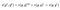# The BLS Signature Scheme — A short intro

Outline:

1. What is a Signature Scheme?
2. Some well-known signature schemes
3. Diffie-Hellman Problem (DHP)
4. Computational DHP vs Decisional DHP
5. Gap Group
6. BLS Signature Scheme

A digital signature scheme is essentially a mathematical setup to prove that a message has been authenticated by the sending party. The signature scheme ensures:

• Message is authenticated (by sender)
• Sender cannot deny sending the message (non-repudiation)
• Message is not tampered with (data integrity)

Diffie and Hellman conjectured the existence of a digital signature scheme in 1976 based on trapdoor one-way functions. (One-way functions have not been proven to exist to date.) Shortly after, the RSA encryption scheme was invented based upon the assumption that the factorization of semi-primes (N=p*q, where p, q are primes) is a difficult problem.

Soon after, many more encryption schemes were invented:

Some prominent encryption schemes in literature and the fundamental reasoning behind their formulation include:

• Boneh — Lynn — Shacham (BLS): Based on the existence of random oracles and the intractability of the computational Diffie-Hellman problem in a gap Diffie-Hellman group.
• ElGamal: Based on the hardness of the discrete logarithm problem.
• ECDSA: Based on the fact that given a point A on an elliptic curve and another point P = n*A, it is difficult to find n.
• Ring signature: Any non-empty subset of a group of n people can sign a message without the identity of any of the participants being leaked. It is a signature scheme built on other schemes.
• RSA: Factorization of numbers (semi-primes in particular) is hard.
• Schnorr Signature: Based on the hardness of the discrete logarithm problem.

With the broad understanding above, we now take a look at the Diffie-Hellman problem.

# Diffie-Hellman Problem (DHP)

Given a multiplicative group G and a generator g, the Diffie-Hellman Problem (DHP) asks whether it is easy to find g^xy given g, g^x, and g^y, where x, y are some randomly chosen integers. Solving the Diffie-Hellman problem (DHP) in many groups has been shown to be almost as hard as the discrete log problem (DLP).

# Computational DHP (CDHP) vs Decisional DHP (DDHP)

At this stage, it is important to make a distinction between the computational DHP and the decisional DHP. The computational DHP involves finding g^xy from g^x and g^y, as described before, whereas the decisional DHP (DDHP) involves distinguishing g^xy from a random group element given g, g^x, g^y. It is trivial to see that the computational DHP is always as difficult as the decisional DHP, but it is unclear to see if one is strictly tougher in general.

# Gap Group

A gap group is a group in which the computational DHP is difficult but the DDHP can be efficiently solved. One particular example is that of non-degenerate, efficiently computable, bilinear pairings. A pairing in cryptography is defined as follows:

We can see that the decisional DHP when a bilinear pairing is defined is easy because given g^x, g^y, g^z, where z = x*y, we can verify whether g^z is different from a random element in the group G as follows:DDHP is easy in a group over which a bilinear pairing is defined

However, the computational DHP is conjectured to be intractable in this instance.

# BLS Signature Scheme

Having given a primer to digital signature schemes and some basic background, the following are the components of the BLS signature scheme:

• Bilinear Pairing e( · , · )
• Elliptic Curve Group elements as signatures

Assumptions:

• Existence of random oracle
• Intractability of CDHP in a gap DH group

The scheme:

• Generate: Select a number x as your private key in the range [0, r-1]. The public key shared is g^x
• Given the private key x and some message m, the signature is given by (H(m))^x
• To verify that the signature is valid, we check that e((H(m))^x,g) = e(H(m), g^x), given g^x, g

Note that the above signature scheme works, because we have a bilinear pairing on a group and we cannot find the private key x given g^x and g.

In future posts, we will go deeper into the use of digital signature schemes in cryptocurrencies and blockchains.

About Chain Intelligence — Based in New York City, Chain Intelligence is a technical consulting group dedicated to quality blockchain research.

Written by

## Chain Intelligence

Written by

#### PhD @ Courant Institute of Mathematical Sciences. Interested in Machine Learning, Cryptography and Blockchain.## More From Medium

Welcome to a place where words matter. On Medium, smart voices and original ideas take center stage - with no ads in sight. Watch
Follow all the topics you care about, and we’ll deliver the best stories for you to your homepage and inbox. Explore
Get unlimited access to the best stories on Medium — and support writers while you’re at it. Just \$5/month. Upgrade